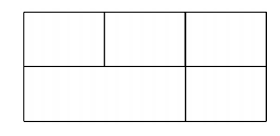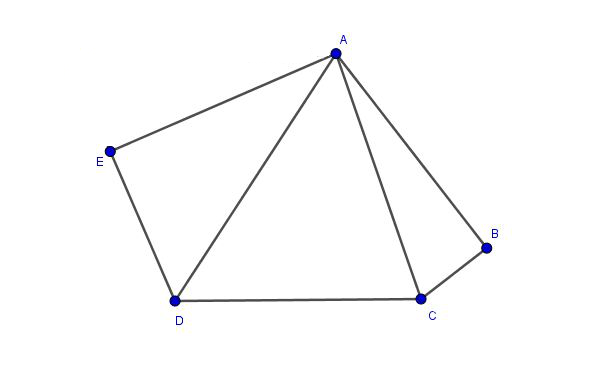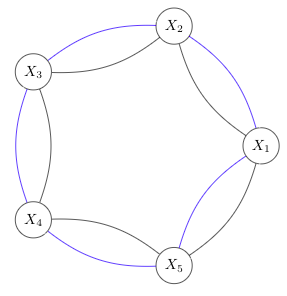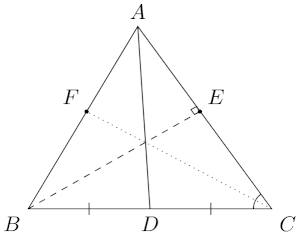# MT #9: Full-syllabus

## Part A: Short-answer type questions

Submission file: Write answers to all the ten questions on a single sheet of paper. Email a picture of your answer sheet. Name the file as PartA.jpg.

For this part, answers must be written without any explanation.

1. Let $$n$$ be a positive integer. Line segments can be drawn parallel to edges of a given rectangle. What is the minimum number of line segments that are required so as to divide the rectangle into exactly $$n$$ subrectangles? The subrectangles need not be of the same dimension.

For example, in order to get 5 subrectangles we need 3 line segments.2. The polynomial $$f(x)=a x^{2018}+b x^{2017}+c x^{2016}$$ has real coefficients less than 2020 and $f\left(\frac{1+\sqrt{3} i}{2}\right)=2015+2019 \sqrt{3} i$ Write the value of $$f(1)$$.

3. Let $$X$$ be a set and $$A, B, C$$ be its subsets. Which of the following is necessarily true?
(a) $$A-(A-B)=B$$
(b) $$A-(B \cup C)=(A-B) \cup(A-C)$$
(c) $$A-(B \cap C)=(A-B) \cap(A-C)$$
(d) $$B-(A-B)=B$$

4. Find the number of functions $$f:\{1,\ldots, 5\} \to \{1,\ldots, 5\}$$ such that $$f(f(x))=x$$ .

5. The number of roots of $$g(x)=5 x^{4}-4 x+1=0$$ in $$[0,1]$$ is
(a) 0
(b) 1
(c) 2
(d) 3

6. Evaluate : $$\lim\limits_{n\to\infty} \displaystyle\int_{0}^{1} \left(1 + \frac{x}{n}\right)^n dx$$

7. Consider a square $$ABCD$$, whose length of a side is $$7$$ units. Now, draw an equilateral triangle $$ABM$$ so that the vertex $$M$$ lies inside the square. The diagonal $$AC$$ meets the triangle at the point $$K$$. Find out the value of $$| CK - KM |$$ .

8. $$2021$$ players numbered $$1, 2, \cdots , 2021$$ took part in a table tennis tournament. Each player played exactly one game against each of the $$2020$$ other players, and no match ended in a draw. Let $$W_k$$ and $$L_k$$ denote the number of matches won and lost respectively by the $$k$$-th player, for $$k = 1, 2, \cdots , 2021$$. Find out which among the following options is correct :
1. $$~~~~\displaystyle\sum_{k=1}^{2021} W^2_k = (2020)^2 - \displaystyle\sum_{k=1}^{2021} L^2_k$$
2. $$~~~~\displaystyle\sum_{k=1}^{2021} W^2_k = \binom{2021}{2} + \displaystyle\sum_{k=1}^{2021} L^2_k$$
3. $$~~~~\displaystyle\sum_{k=1}^{2021} W^2_k = (2020)^2 + \displaystyle\sum_{k=1}^{2021} L^2_k$$
4. $$~~~~\displaystyle\sum_{k=1}^{2021} W^2_k = \displaystyle\sum_{k=1}^{2021} L^2_k$$
5. $$~~~~\displaystyle\sum_{k=1}^{2021} W^2_k = 2021 + \displaystyle\sum_{k=1}^{2021} L^2_k$$

9. Let $$\Lambda$$ be the set of all $$n \in \mathbb{N}$$ such that both the numbers $$(n+3)$$, and $$(n^2 + 3n +3)$$ are perfect cubes. Find the cardinality of the set $$\Lambda$$.

10. Consider $$ABCDE$$ to be a convex pentagon such that $$AB = AE = CD = 1$$, $$\angle ABC = \angle DEA = 90^\circ$$ , and $$BC + DE = 1$$. Find the area of the pentagon.## Part B: Subjective questions

Submission files: Each question in this part must be answered on a page of its own. Name the files as B1.jpg, B2.jpg, etc. In case you have multiple files for the same question, say B4, name the corresponding files as B4-1.jpg, B4-2.jpg, etc.

Clearly explain your entire reasoning. No credit will be given without reasoning. Partial solutions may get partial credit.

B1. Find all real $$x$$ for which the equation holds: $4^{x}+9^{x}+36^{x}+\sqrt{\frac{1}{2}-2 x^{2}}=1$

B2. Consider a set of $$n$$ islands $$X_1, \ldots , X_n$$ arranged in a circle. Assume $$n\geq 2$$. There are two bridges between each pair of neighboring islands. For example, the situation for $$n=5$$ is shown below:(a) Suppose we start from island $$X_1$$ and cross the $$2n$$ bridges exactly once. Show that we will return to $$X_1$$ at the end of the journey. $$\;\;$$ [2 marks]
(b) Stating from the island $$X_1$$, how many ways one can cross the $$2n$$ bridges so that each bridge is crossed exactly once? $$\;\;$$ [8 marks]

B3. Consider the sequence of natural numbers $$a_1,a_2,\ldots,a_n$$ defined as follows:

• $$a_1=2$$.
• For each positive number $$n$$, $$a_{n+1} = a_n +$$ product of the prime factors of $$a_n$$.
The first eight numbers of the sequence are: $$2, 4, 6, 12, 18, 24, 30 \text{ and } 60$$.
(a) Does the number 210 appear in the sequence? $$\;\;$$ [1 mark]
(b) Prove that the product of the first 2021 primes appears in the sequence. $$\;\;$$ [9 marks]

B4. $$A B C$$ is an acute triangle with $$B C=4$$ and $$A C=5$$. Let $$D$$ be the midpoint of $$BC$$. $$BE$$ is the altitude to $$AC$$. $$CF$$ is the angle bisector of $$\angle B C A$$. If $$A D, B E$$, and $$C F$$ meet at a single point, compute the length of $$BE$$.B5. Consider a real polynomial of the form $$p(x) = ax^4 + bx^2+c$$. We are given that $$0\leq p(x)\leq 1$$ for all $$0 \leq x \leq 1$$.

1. Show that the above condition implies that $$a\leq 4$$. $$\;\;$$ [7 marks]
2. Find a real polynomial with $$a=4$$ that satisfies the given condition. $$\;\;$$ [3 marks]

B6. Let $$\{a_k\}_{k \in \mathbb{N} }$$ be a strictly increasing sequence of positive integers. Define the sequence $$\{s_n\}_{n \in \mathbb{N}}$$ as $s_n=\sum_{k=1}^{n}\frac{1}{[a_k,a_{k+1}]}$ where $$[a_k,a_{k+1}]$$ is the least common multiple (i.e. LCM) of $$a_k$$ and $$a_{k+1}$$.

1. Show that the sequence $$\{s_n\}_{n \in \mathbb{N}}$$ is bounded above. $$\;\;$$[8 marks]
2. Prove that $$\{s_n\}_{n \in \mathbb{N}}$$ converges.$$\;\;$$[2 marks]